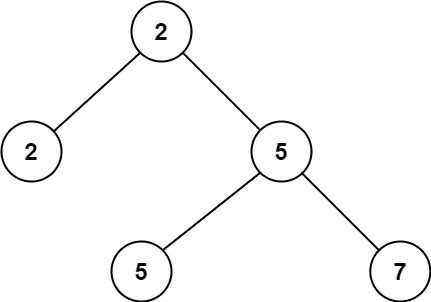# Leetcode 671 - Second Minimum Node In a Binary Tree

Note:

• The root is the min val in the tree.
• Set up secondMin = Infinity.
• Traverse all nodes, and compare with val < secondMin && val > Min.

Question:

Given a non-empty special binary tree consisting of nodes with the non-negative value, where each node in this tree has exactly two or zero sub-node. If the node has two sub-nodes, then this node’s value is the smaller value among its two sub-nodes. More formally, the property root.val = min(root.left.val, root.right.val) always holds.

Given such a binary tree, you need to output the second minimum value in the set made of all the nodes’ value in the whole tree.

If no such second minimum value exists, output -1 instead.

Example:Code: